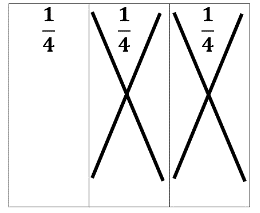# Access Point #: MAFS.4.NF.2.AP.3c

Solve word problems involving addition and subtraction of fractions with like denominators (2, 3, 4 or 8).
Clarifications:

#### Essential Understandings

Concrete:

• Match the vocabulary in a word problem to an action.
• Use manipulatives to model the context of the word problem.
• Count to find the answer.
• To add, use fraction manipulatives (each piece may be labeled with the corresponding unit fraction) to model each fraction and join them to find the sum (e.g.,1/4 + 2/4 = 3/4).
• To subtract, use fraction manipulatives (each piece may be labeled with the corresponding unit fraction) to model the first fraction in the expression and remove manipulatives that represent the fraction being subtracted (e.g., 3/4 – 2/4 = 1/4 ).Representation:
• Create a pictorial representation of the word problem.
• Use context clues to interpret the concepts, symbols, and vocabulary for addition and subtraction.
• To add, use a visual representation of a whole divided into equal pieces (each piece may be labeled with the corresponding unit fraction). Shade each unit to represent the fractions in the expression and count the shaded units to find the sum.
• To subtract, use a visual representation of the first fraction in the expression. Cross out the piece(s) that represent the fraction being subtracted. Count the remaining piece(s) to find the remainder.
General Information
Number: MAFS.4.NF.2.AP.3c
Category: Access Points
Cluster: Build fractions from unit fractions by applying and extending previous understandings of operations on whole numbers. (Major Cluster)

Clusters should not be sorted from Major to Supporting and then taught in that order. To do so would strip the coherence of the mathematical ideas and miss the opportunity to enhance the major work of the grade with the supporting clusters.

## Related Standards

This access point is an alternate version of the following benchmark(s).

## Related Courses

This access point is part of these courses.
5012015: Foundational Skills in Mathematics 3-5

## Related Resources

Vetted resources educators can use to teach the concepts and skills in this access point.

## Element Cards

Element Cards are available to assist in planning for instruction. They are designed to promote understanding of how students move toward the academic standards. Element Cards contain one or more access points, essential understandings, suggested instructional strategies and suggested supports.

Type: Element Cards

## Professional Development

Fractions and Decimals Content Module :

Content Modules provide explanations and examples of the concepts contained in the academic standards that may be difficult to teach or unfamiliar to special education teachers. They promote an understanding of the concepts so that a teacher can begin to plan how to teach the concepts to students. Content Modules contain potential adaptations, modifications and ideas for Universal Design for Learning.

Type: Professional Development

## Teaching Idea

Curriculum Resource Guide: Fractions and Decimals:

complete file

Type: Teaching Idea

## Student Resources

Vetted resources students can use to learn the concepts and skills in this access point.

## Parent Resources

Vetted resources caregivers can use to help students learn the concepts and skills in this access point.